# Perimeter Of A Triangle - Definition with Examples

The Complete K-5 Math Learning Program Built for Your Child

• 40 Million Kids

Loved by kids and parent worldwide

• 50,000 Schools

Trusted by teachers across schools

• Comprehensive Curriculum

Aligned to Common Core

## Perimeter of a Triangle

A perimeter of a shape is the length of its boundary or the total length around the shape. The perimeter of a 2D closed shape = sum of all its side.

Triangle

A triangle is a polygon having three sides. In other words, it is a closed 2D shape having three straight sides.In the given triangle, a, b and c are the sides of the triangle.

Perimeter of a triangle

The perimeter of a triangle is the sum of all its three sides. We can work out the perimeter using the following formula:

 Perimeter of a triangle = sum of all three sides

If a, b and c are the sides of the triangle, then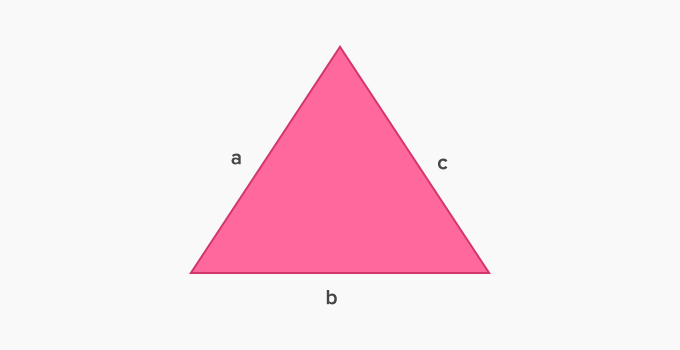Perimeter of triangle = a + b + c

Also, the unit of a triangle's perimeter is the same as the units of the lengths of its sides. In case, the units of lengths of its sides are different, then first convert them into the same unit.

Example 1: Find the perimeter of the given triangle.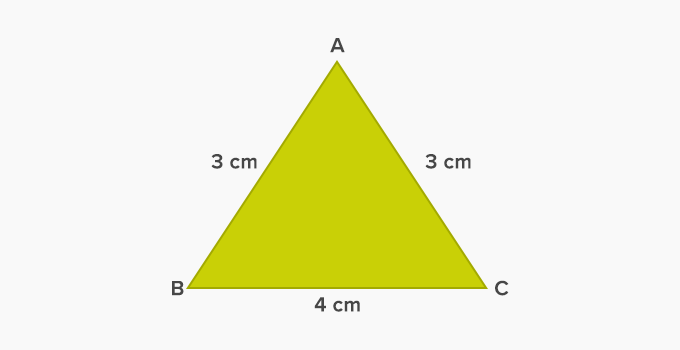Here, sides of the triangle are AB = 3 cm, BC = 4 cm and AC = 3 cm

Perimeter of triangle = sum of all three sides

Therefore, the perimeter of the given triangle = 3 cm + 4 cm + 3 cm = 10 cm

Perimeter of an isosceles triangle: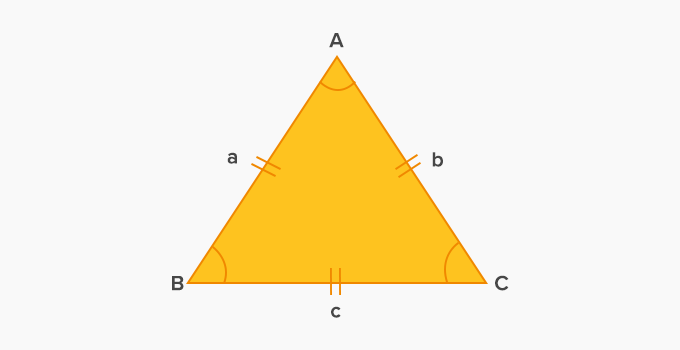Perimeter of an isosceles triangle = a + b + c

Here sides AB and AC are equal length so we can write the above formula as given below.

Perimeter of an isosceles triangle = a + a + c or  b + b + c

This can also be written as 2a + c or 2b + c or 2✕ equal side + c

Perimeter of an equilateral triangle: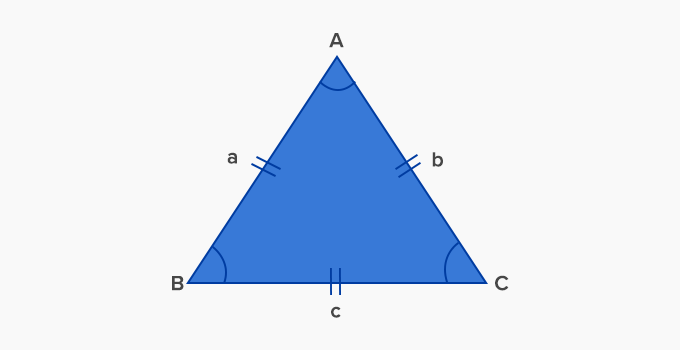Perimeter of an equilateral triangle = a + b + c

As we know sides of an equilateral are equal in length.

So, the perimeter of an equilateral triangle = a + a + a= 3a

This formula can also be written as:

Perimeter of an equilateral triangle= 3 ✕ side

Using perimeter of a triangle in our everyday life

Following are the examples where we use the perimeter of a triangle in our daily life:

• The boundary of a triangular

• The boundary of a triangular hut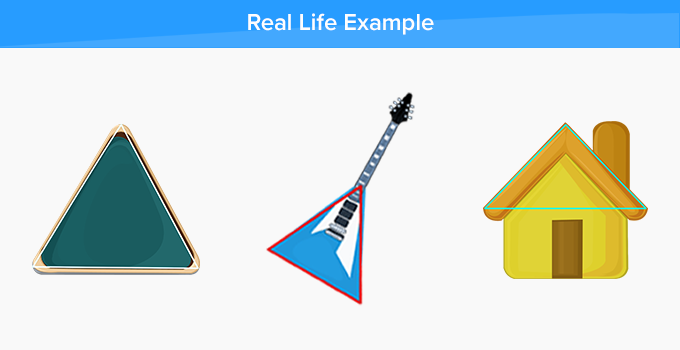Fun Facts  The word perimeter is made up of two Greek words – “peri” which means around and “metron” which means measure.

Won Numerous Awards & Honors